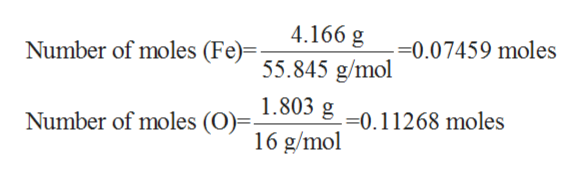# in a reaction involving iron, Fe, and oxygen, O. it was determined that 4.166 grams of iron reacted with 1.803 grams of oxygen. form this information, determine the empirical formula of the compound that resulted.

Question
86 views

in a reaction involving iron, Fe, and oxygen, O. it was determined that 4.166 grams of iron reacted with 1.803 grams of oxygen. form this information, determine the empirical formula of the compound that resulted.

check_circle

Step 1

The term empirical formula in chemistry refers to the simplest positive integer ratio of all the atoms which are present in the given compound.

Step 2

The term number of moles refers to the ratio of mass to the molar mass of the given compound. The chemical formula is as shown below:

Step 3

In the given problem, it has been given that the mass of Fe which reacts with oxygen is 4.166 g and the mass of oxygen is given to be 1.803 g.

To determin...help_outlineImage Transcriptionclose4.166 g 55.845 g/mol 1.803 g -0.11268 moles 16 g/mol Number of moles (Fe)- =0.07459 moles Number of moles (O)- fullscreen

### Want to see the full answer?

See Solution

#### Want to see this answer and more?

Solutions are written by subject experts who are available 24/7. Questions are typically answered within 1 hour.*

See Solution
*Response times may vary by subject and question.
Tagged in

### Inorganic Chemistry